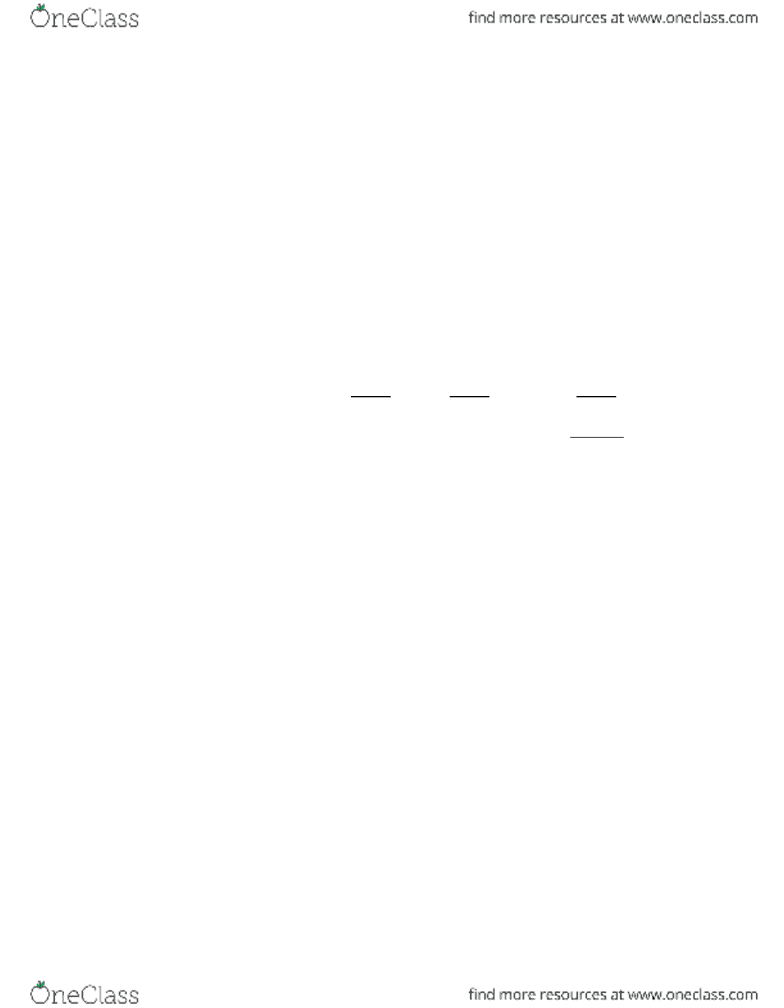Textbook Notes (270,000)
CA (160,000)
York (10,000)
ADMS (1,000)
Chapter 7

# ADMS 3530 Chapter Notes - Chapter 7: American Electric Power, Net Income, Efficient-Market Hypothesis

Department
Administrative Studies
Course Code
ADMS 3530
Professor
Lois King
Chapter
7

This preview shows pages 1-3. to view the full 23 pages of the document.Brealey 5CE
Solutions to Chapter 7
1. No. The dividend discount model allows for the fact that firms may not currently
pay dividends. As the market matures, and Research in Motion’s growth
opportunities moderate, investors may justifiably believe that Research in Motion
will enjoy high future earnings and will pay dividends then. The stock price today
can still reflect the present value of the expected per share stream of dividends.
2. Dividend yield = Expected dividend/Price = DIV1/P0
So: P0 = DIV1/dividend yield
P0 = \$2.4/.08 = \$30
3. a. The typical preferred stock pays a level perpetuity of dividends. The expected
dividend next year is the same as this year’s dividend, \$8. Thus the dividend
growth rate is zero and the price today is:
P0 = D1/r = 8/.12 = \$66.67
b. The expected dividend in two years is this year’s dividend, \$8.
P1= D2/r = 8/.12 = \$66.67
c. Dividend yield = \$8/\$66.67 = .12 =12%
Expected capital gains = 0
Expected rate of return = 12%
4. r = DIV1/P0 + g = 8% + 5% = 13%
5. The value of a common stock equals the present value of dividends received out
to the investment horizon, plus the present value of the forecast stock price at the
horizon. But the stock price at the horizon date depends on expectations of
dividends from that date forward. So even if an investor plans to hold a stock for
only a year for two, the price ultimately received from another investor depends
on dividends to be paid after the date of purchase. Therefore, the stock’s present
value is the same for investors with different time horizons.
6. a. P0 = r = + g
r = \$3/\$30+ .04 = .14 = 14%
b. P0 = 3.00/(.165 .04) = \$24
7-1
Copyright © 2012 McGraw-Hill Ryerson Limited

Only pages 1-3 are available for preview. Some parts have been intentionally blurred.7. The dividend yield is defined as the annual dividend (or the annualized current
dividend) divided by the current price. The current annual dividend is
(\$2 × 4) = \$8 and the dividend yield is:
DIV1/P0 = .048 \$8/ P0 = .048 P0 = \$8/.048 = \$166.7
To work with the quarterly dividend, divide the dividend yield by 4 and repeat the
above steps:
Quarterly DIV/P0 = .048/4 = .012 \$2/ P0 = .012 P0 = \$2/.012 = \$166.7
8. Weak, semi-strong, strong, fundamental, technical
9. True. The search for information and insightful analysis is what makes investor
assessments of stock values as reliable as possible. Since the rewards accrue to the
investors who uncover relevant information before it is reflected in stock prices,
competition among these investors means that there is always an active search on for
mispriced stocks.
10. Two such behavioral biases are alluded to in the text.
a.) Attitudes toward risk: Psychologists have observed the tendency of investors to be
particularly averse to the possibility of incurring losses. Consequently, when investors
sustain a loss they tend to exercise excessive conservatism in their subsequent
investment decisions to avoid worsening their deficit. Conversely, when investors have
secured a gain they are more eager to take on risky bets because they take comfort in
their cushion of profit. This behavioral bias has been implicated as a cause of stock
market “bubbles”: a phenomenon where market prices far exceed intrinsic firm values.
b.) Beliefs about probabilities: This bias is twofold. Firstly, investors tend to look at
previous periods and assume that future market fluctuations will follow suit. This is
incorrect since stock prices follow a random walk and ought to be independent of
previous market movements. To further compound their error, investors tend to be
myopic and place greater emphasis on recent market developments; as such, they
overlook valuable information from the distant past (i.e. market reactions to long-term
economic cycles). Secondly, investors tend to fall prey to overconfidence. When
investors profit in the market they wrongly attribute their success to skill rather than
mere luck. Such excessive optimism may cause prices to greatly deviate from intrinsic
share values.
7-2
Copyright © 2012 McGraw-Hill Ryerson Limited

Only pages 1-3 are available for preview. Some parts have been intentionally blurred.11. a. DIV1 = \$1 × 1.04 = \$1.04
DIV2 = \$1 × 1.042 = \$1.0816
DIV3 = \$1 × 1.043 = \$1.1249
b. P0 = DIV1/(r g) = = \$13
c. P3 = DIV4/(r g) = = \$14.6237
Note: the number of decimal places carried affects the solution. If you used this
formula the answer would be:
P3 = DIV4/(r g) = = \$14.623232, about \$14.6232
d. Your payments are:
Year 1 Year 2 Year 3
DIV 1.04 1.0816 1.1249
Sales Price 14.6237
Total cash flow 1.04 1.0816 15.7486
PV of cash flow .9286 .8622 11.2095
Sum of PV = \$13.00, the same as your answer to (b).
12. Dividend growth rate, g = return on equity × plowback ratio:
g = .15 × .40 = .06
r = + g = + .06 = .16 = 16%
13. a. P0 = = (3 x 1.05) / (0.15 - 0.05) = \$31.50
b. P0 = (3 x 1.05) / (0.12 – 0.05) = \$45
The lower discount rate makes the present value of future dividends higher,
raising the value of the stock.
c. P0 = (3 x (1 – .05) / (.15 – (-.05)) = \$14.25
The price is the present value of the future dividends before they are eroded by
the negative growth rate.
7-3
Copyright © 2012 McGraw-Hill Ryerson Limited
###### You're Reading a Preview

Unlock to view full version